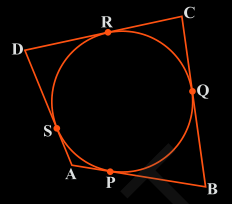Guru

# A quadrilateral ABCD is drawn to circumscribe a circle (see Fig. 10.12). Prove that AB + CD = AD + BC Q.8

• 0

The question from class 10th ncert book of exercise 10.2 of question no.8 of circles chapter, how i solve this question in easy way i think it is very important for class 10th, A quadrilateral ABCD is drawn to circumscribe a circle (see Fig. 10.12). Prove that AB + CD = AD + BC

Share

1. The figure given is:From this figure we can conclude a few points which are:

(i) DR = DS

(ii) BP = BQ

(iii) AP = AS

(iv) CR = CQ

Since they are tangents on the circle from points D, B, A, and C respectively.

Now, adding the LHS and RHS of the above equations we get,

DR+BP+AP+CR = DS+BQ+AS+CQ

By rearranging them we get,

(DR+CR) + (BP+AP) = (CQ+BQ) + (DS+AS)

By simplifying,